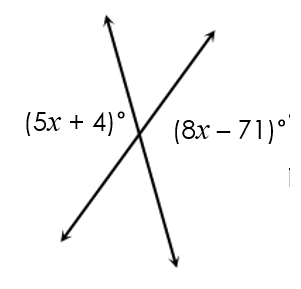Chapter 1 summative assignment 1
Last Name *
Period *
Identify *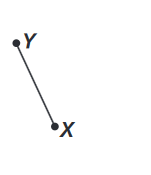Identify the INCORRECT way to name this angle *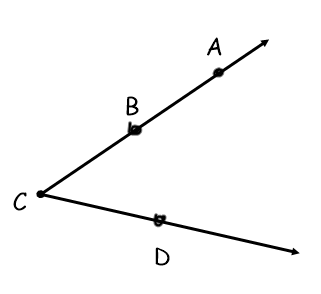Identify the vertex of the marked angle *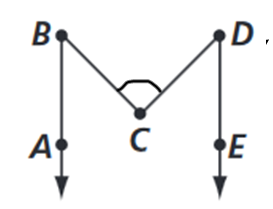Identify *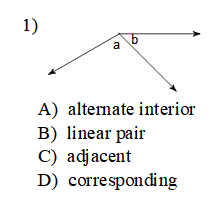Identify *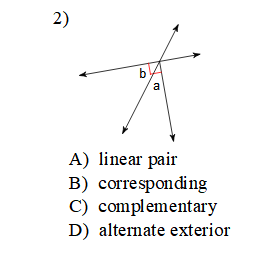Two lines that intersect and form a right angle are called... *
Find b. *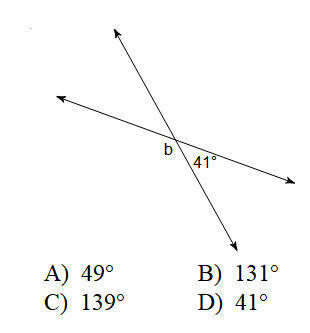Find x. *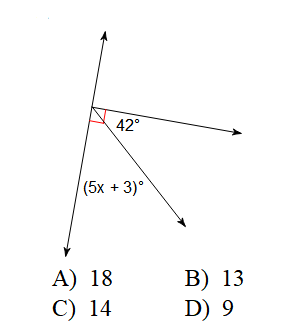Label correctly and Find the missing angle *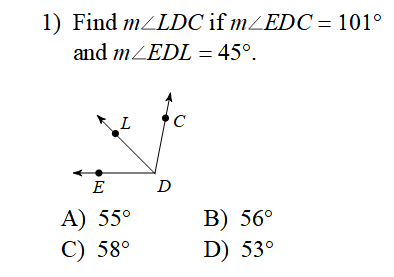Find x. *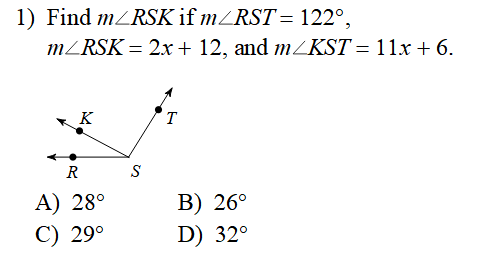Two angles are supplementary. one angle is 3x + 18 and the other is 5x -10. Find x *
Angle A is congruent to angle B. If these angles are complementary, What is the measure of angle B? *
Find x. *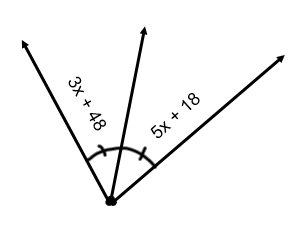Solve for x. *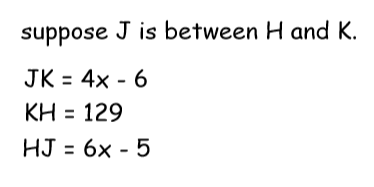Let B be the midpoint of AC. If AB = 2x + 21, and BC = 5x - 9. What is x? *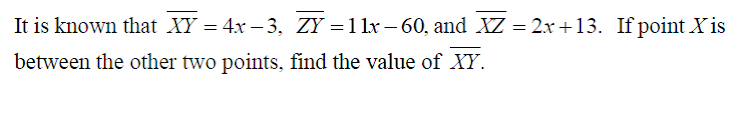Find angle b. *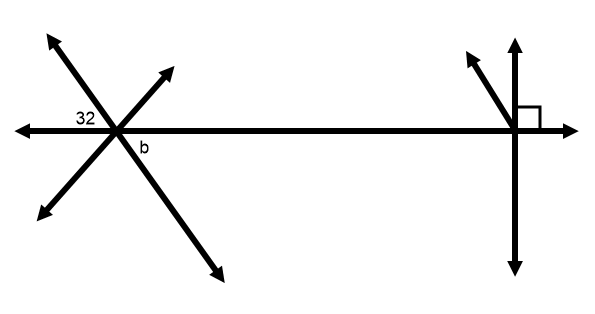Find m<DFE *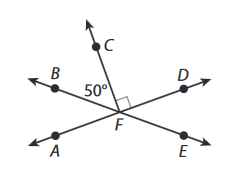Find m<AFE *Find angle x. *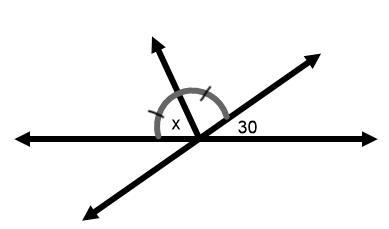Which three points below are collinear? *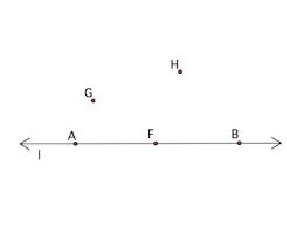Pick the best description for angles 1 and 2 *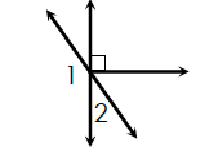Solve for x. *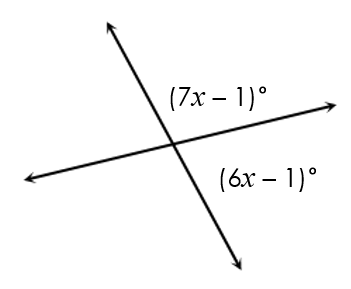Solve for x. *